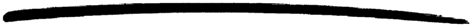# string

string

### MODULE SUMMARY

String Processing Functions

### DESCRIPTION

This module contains functions for string processing.

### EXPORTS

Types:

String = string()
Length = integer()

Returns the number of characters in the string.

Types:

String1 = String2 = string()

Tests whether two strings are equal. Returns true if they are, otherwise false.

Types:

String1 = String2 = String3 = string()

Concatenates two strings to form a new string. Returns the new string.

Types:

String = string()
Character = char()
Index = integer()

Returns the index of the first/last occurrence of Character in String. 0 is returned if Character does not occur.

Types:

String = SubString = string()
Index = integer()

Returns the position where the first/last occurrence of SubString begins in String. 0 is returned if SubString does not exist in String. For example:

```> string:str(" Hello Hello World World ", "Hello World").
8
```

Types:

String = Chars = string()
Length = integer()

Returns the length of the maximum initial segment of String, which consists entirely of characters from (not from) Chars.

For example:

```> string:span("\t    abcdef", " \t").
5
> string:cspan("\t    abcdef", " \t").
0
```

Types:

String = SubString = string()
Start = Length = integer()

Returns a substring of String, starting at the position Start, and ending at the end of the string or at length Length.

For example:

```> substr("Hello World", 4, 5).
"lo Wo"
```

Types:

String = SeparatorList = string()
Tokens = [string()]

Returns a list of tokens in String, separated by the characters in SeparatorList.

For example:

```> tokens("abc defxxghix jkl", "x ").
["abc", "def", "ghi", "jkl"]
```

Types:

StringList = [string()]
Separator = string()

Returns a string with the elements of StringList seperated by the string in Seperator.

For example:

```> join(["one", "two", "three"], ", ").
"one, two, three"
```

Types:

Character = char()
Number = integer()
String = string()

Returns a string consisting of Number of characters Character. Optionally, the string can end with the string Tail.

Types:

String = Copies = string()
Number = integer()

Returns a string containing String repeated Number times.

Types:

String = string()
Character = char()
Count = integer()

Returns the number of words in String, separated by blanks or Character.

For example:

```> words(" Hello old boy!", \$o).
4
```

Types:

String = Word = string()
Character = char()
Number = integer()

Returns the word in position Number of String. Words are separated by blanks or Characters.

For example:

```> string:sub_word(" Hello old boy !",3,\$o).
"ld b"
```

Types:

String = Stripped = string()
Direction = left | right | both
Character = char()

Returns a string, where leading and/or trailing blanks or a number of Character have been removed. Direction can be left, right, or both and indicates from which direction blanks are to be removed. The function strip/1 is equivalent to strip(String, both).

For example:

```> string:strip("...Hello.....", both, \$.).
"Hello"
```

Types:

String = Left = string()
Character = char
Number = integer()

Returns the String with the length adjusted in accordance with Number. The left margin is fixed. If the length(String) < Number, String is padded with blanks or Characters.

For example:

```> string:left("Hello",10,\$.).
"Hello....."
```

Types:

String = Right = string()
Character = char
Number = integer()

Returns the String with the length adjusted in accordance with Number. The right margin is fixed. If the length of (String) < Number, String is padded with blanks or Characters.

For example:

```> string:right("Hello", 10, \$.).
".....Hello"
```

Types:

String = Centered = string()
Character = char
Number = integer()

Returns a string, where String is centred in the string and surrounded by blanks or characters. The resulting string will have the length Number.

Types:

String = SubString = string()
Start = Stop = integer()

Returns a substring of String, starting at the position Start to the end of the string, or to and including the Stop position.

For example:

```sub_string("Hello World", 4, 8).
"lo Wo"
```

Types:

String = string()
Float = float()
Rest = string()
Reason = no_float | not_a_list

Argument String is expected to start with a valid text represented float (the digits being ASCII values). Remaining characters in the string after the float are returned in Rest.

Example:

```          > {F1,Fs} = string:to_float("1.0-1.0e-1"),
> {F2,[]} = string:to_float(Fs),
> F1+F2.
0.900000
> string:to_float("3/2=1.5").
{error,no_float}
> string:to_float("-1.5eX").
{-1.50000,"eX"}

```

Types:

String = string()
Int = integer()
Rest = string()
Reason = no_integer | not_a_list

Argument String is expected to start with a valid text represented integer (the digits being ASCII values). Remaining characters in the string after the integer are returned in Rest.

Example:

```          > {I1,Is} = string:to_integer("33+22"),
> {I2,[]} = string:to_integer(Is),
> I1-I2.
11
> string:to_integer("0.5").
{0,".5"}
> string:to_integer("x=2").
{error,no_integer}

```

Types:

String = Result = string()
Char = CharResult = integer()

The given string or character is case-converted. Note that the supported character set is ISO/IEC 8859-1 (a.k.a. Latin 1), all values outside this set is unchanged

### Notes

Some of the general string functions may seem to overlap each other. The reason for this is that this string package is the combination of two earlier packages and all the functions of both packages have been retained.

Note

Any undocumented functions in string should not be used.

stdlib 1.15
Copyright © 1991-2007 Ericsson AB